# C ObjectOriented Programming Classes and Objects Template classes

• Slides: 31C++: Object-Oriented Programming • • • Classes and Objects Template classes Operator Overloading Inheritance Polymorphism CS 103 1The Evolution of The Notion of Object • In C, a struct models what a thing has/is (i. e. , the data, also called the characteristics), but not what it does (its behavior, represented by functions). • The functions are outside and separate from structs. • In C++, the characteristics and behavior are integrated into a single structure, called object. • The data type of an object is the class of the object • The packaging of the data and the functions into a class type is called data encapsulation. CS 103 2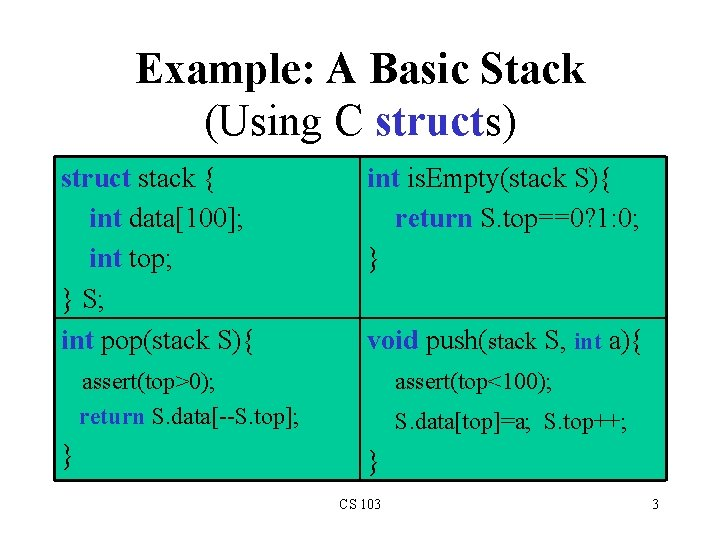Example: A Basic Stack (Using C structs) struct stack { int data; int top; } S; int pop(stack S){ int is. Empty(stack S){ return S. top==0? 1: 0; } void push(stack S, int a){ assert(top>0); return S. data[--S. top]; } assert(top<100); S. data[top]=a; S. top++; } CS 103 3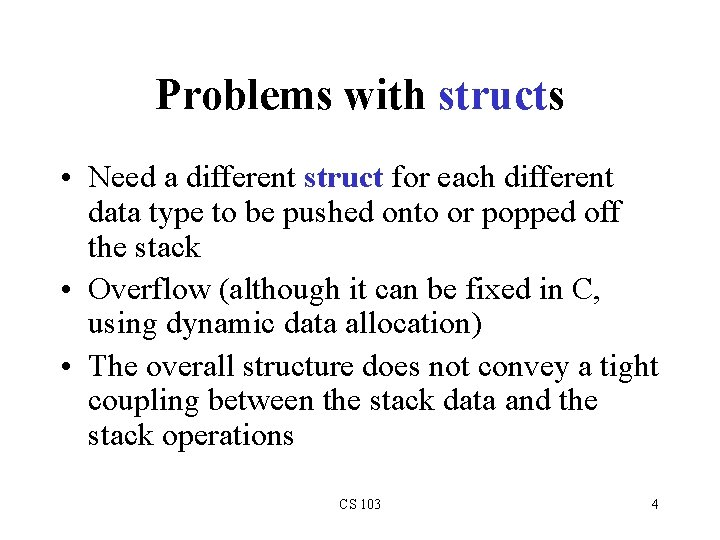Problems with structs • Need a different struct for each different data type to be pushed onto or popped off the stack • Overflow (although it can be fixed in C, using dynamic data allocation) • The overall structure does not convey a tight coupling between the stack data and the stack operations CS 103 4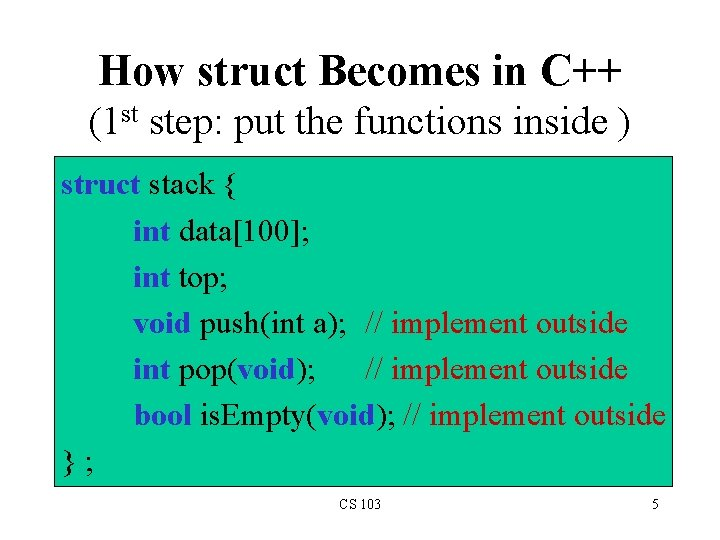How struct Becomes in C++ (1 st step: put the functions inside ) struct stack { int data; int top; void push(int a); // implement outside int pop(void); // implement outside bool is. Empty(void); // implement outside }; CS 103 5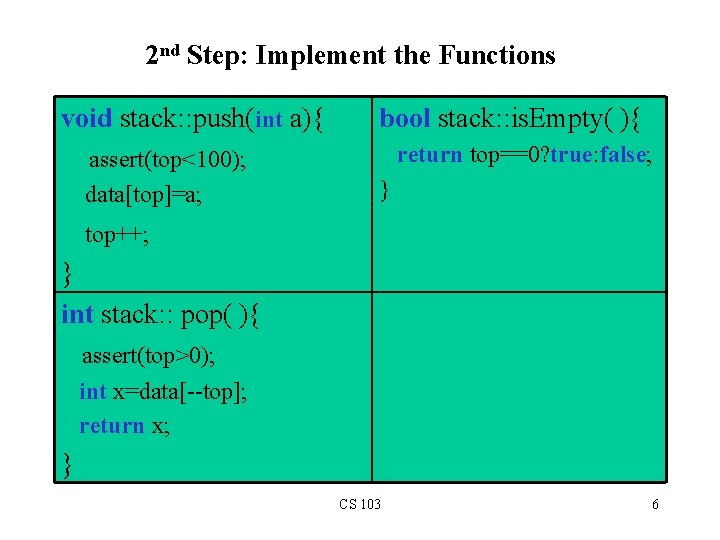2 nd Step: Implement the Functions void stack: : push(int a){ assert(top<100); data[top]=a; bool stack: : is. Empty( ){ return top==0? true: false; } top++; } int stack: : pop( ){ assert(top>0); int x=data[--top]; return x; } CS 103 6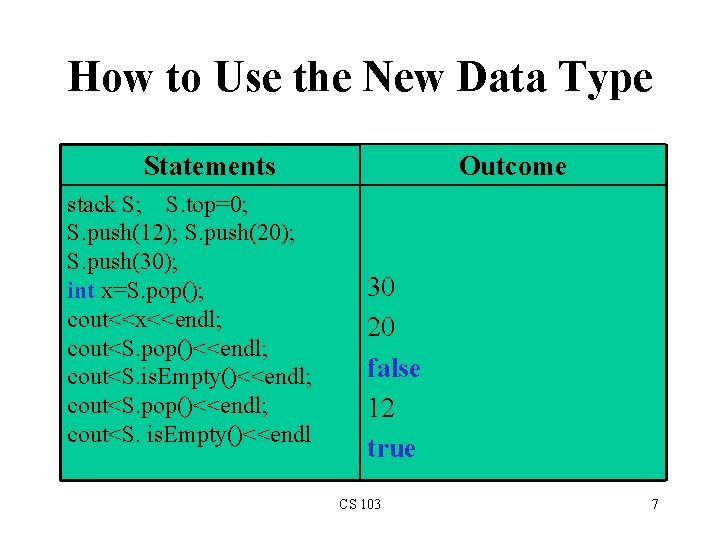How to Use the New Data Type Statements stack S; S. top=0; S. push(12); S. push(20); S. push(30); int x=S. pop(); cout<<x<<endl; cout<S. pop()<<endl; cout<S. is. Empty()<<endl Outcome 30 20 false 12 true CS 103 7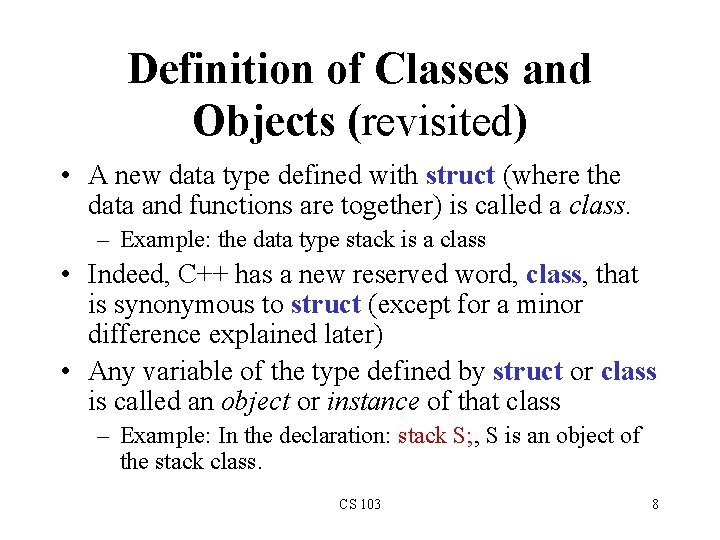Definition of Classes and Objects (revisited) • A new data type defined with struct (where the data and functions are together) is called a class. – Example: the data type stack is a class • Indeed, C++ has a new reserved word, class, that is synonymous to struct (except for a minor difference explained later) • Any variable of the type defined by struct or class is called an object or instance of that class – Example: In the declaration: stack S; , S is an object of the stack class. CS 103 8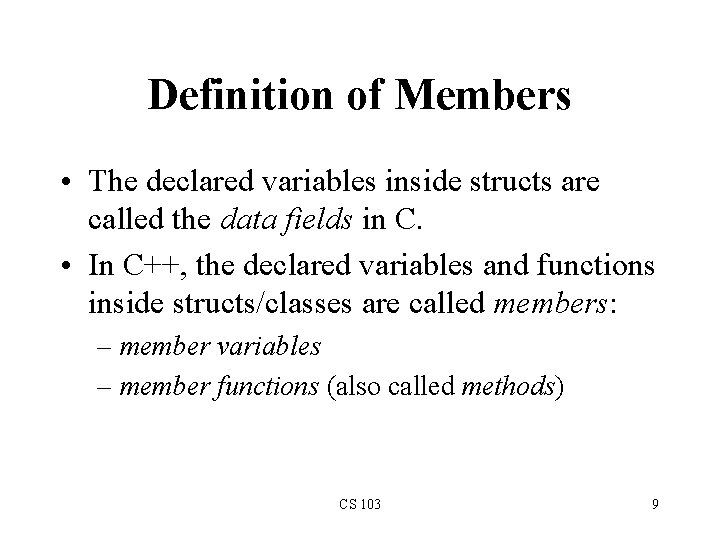Definition of Members • The declared variables inside structs are called the data fields in C. • In C++, the declared variables and functions inside structs/classes are called members: – member variables – member functions (also called methods) CS 103 9Data Hiding: Context • Note that users of a stack object can access directly the members data[] and top • For example, a user can do: S. top -= 3; • But notice that the 3 stack operations are all the users need. They do need to access top directly • Also, this free access can cause problems: – If a user is not careful or is malicious, such a direct access to S. top can invalidate the stack operations’ outcome – It also limits the implementer from evolving/modifying the implementation of the data type CS 103 10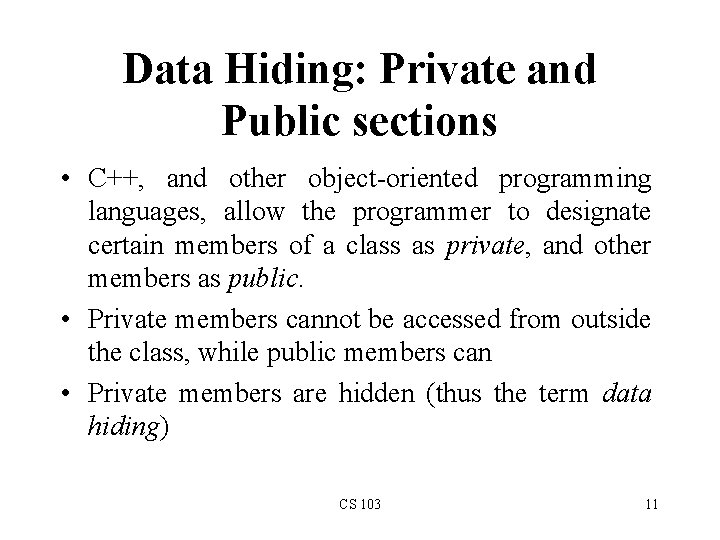Data Hiding: Private and Public sections • C++, and other object-oriented programming languages, allow the programmer to designate certain members of a class as private, and other members as public. • Private members cannot be accessed from outside the class, while public members can • Private members are hidden (thus the term data hiding) CS 103 11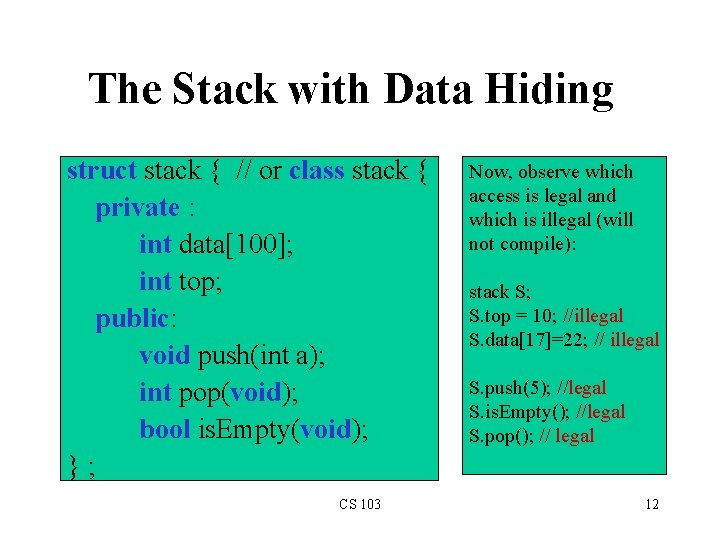The Stack with Data Hiding struct stack { // or class stack { private : int data; int top; public: void push(int a); int pop(void); bool is. Empty(void); }; CS 103 Now, observe which access is legal and which is illegal (will not compile): stack S; S. top = 10; //illegal S. data=22; // illegal S. push(5); //legal S. is. Empty(); //legal S. pop(); // legal 12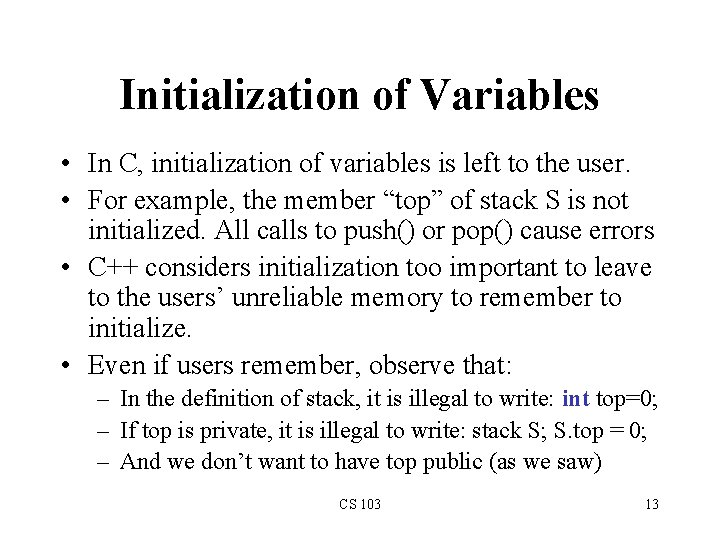Initialization of Variables • In C, initialization of variables is left to the user. • For example, the member “top” of stack S is not initialized. All calls to push() or pop() cause errors • C++ considers initialization too important to leave to the users’ unreliable memory to remember to initialize. • Even if users remember, observe that: – In the definition of stack, it is illegal to write: int top=0; – If top is private, it is illegal to write: stack S; S. top = 0; – And we don’t want to have top public (as we saw) CS 103 13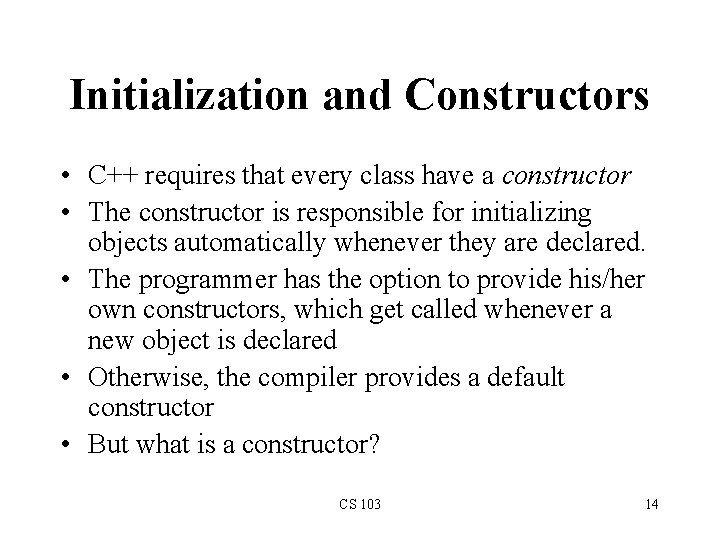Initialization and Constructors • C++ requires that every class have a constructor • The constructor is responsible for initializing objects automatically whenever they are declared. • The programmer has the option to provide his/her own constructors, which get called whenever a new object is declared • Otherwise, the compiler provides a default constructor • But what is a constructor? CS 103 14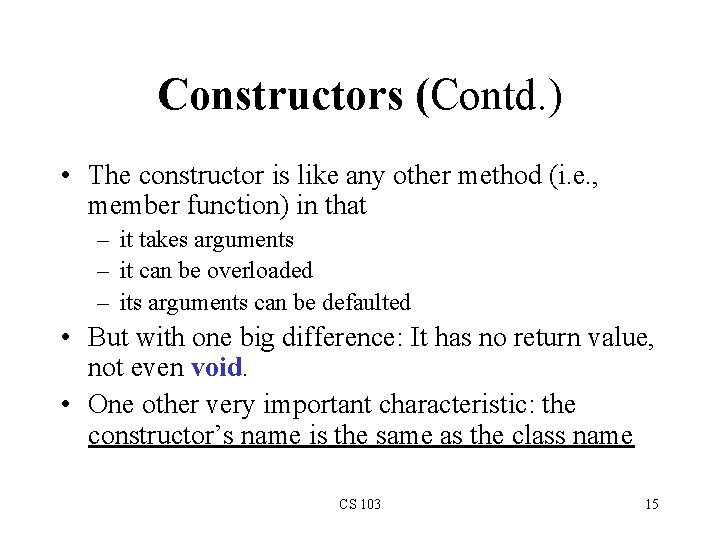Constructors (Contd. ) • The constructor is like any other method (i. e. , member function) in that – it takes arguments – it can be overloaded – its arguments can be defaulted • But with one big difference: It has no return value, not even void. • One other very important characteristic: the constructor’s name is the same as the class name CS 103 15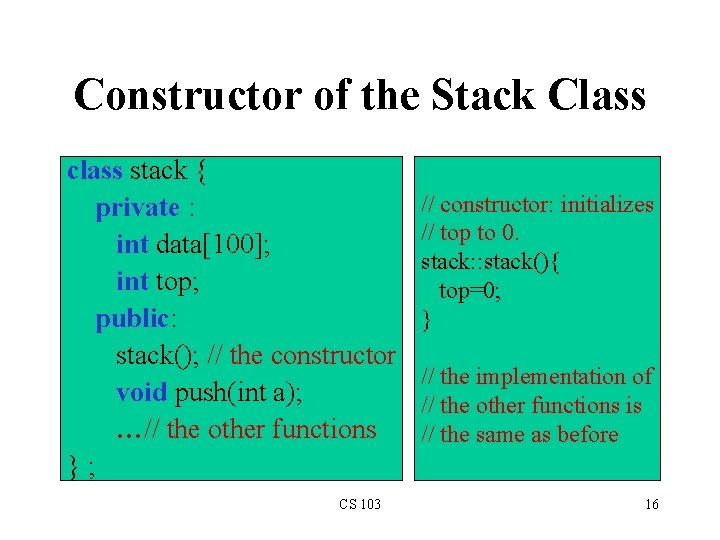Constructor of the Stack Class class stack { private : int data; int top; public: stack(); // the constructor void push(int a); …// the other functions }; CS 103 // constructor: initializes // top to 0. stack: : stack(){ top=0; } // the implementation of // the other functions is // the same as before 16How to Create Objects Using Constructors • Example: stack S(); • This creates S as a stack, and initializes its member “top” to 0. • Now, we can start to call S. push(int), S. pop(), and S. is. Empty() without problems. CS 103 17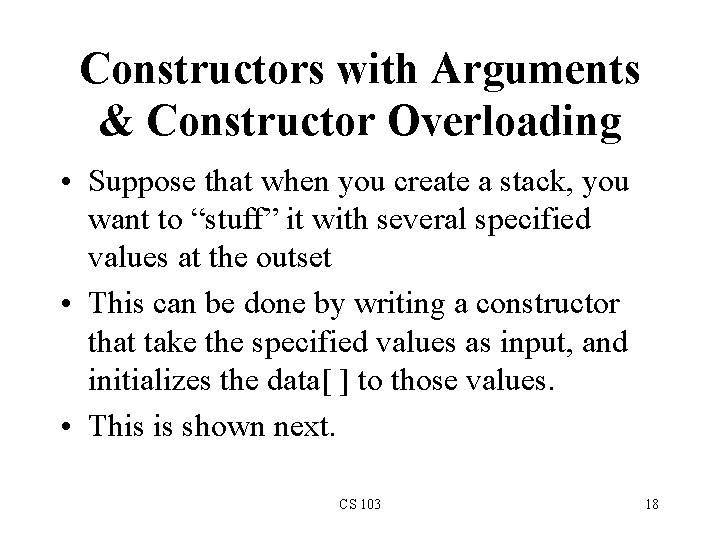Constructors with Arguments & Constructor Overloading • Suppose that when you create a stack, you want to “stuff” it with several specified values at the outset • This can be done by writing a constructor that take the specified values as input, and initializes the data[ ] to those values. • This is shown next. CS 103 18class stack { private : int data; int top; public: stack(); stack(int a[], int n); //other functions }; //constructor: initializes data to a stack: : stack(int a[], int n){ for(top=0; top<n && top<100; top++) data[top]=a[top]; } // constructor: initializes top to 0. stack: : stack(){ top=0; } CS 103 19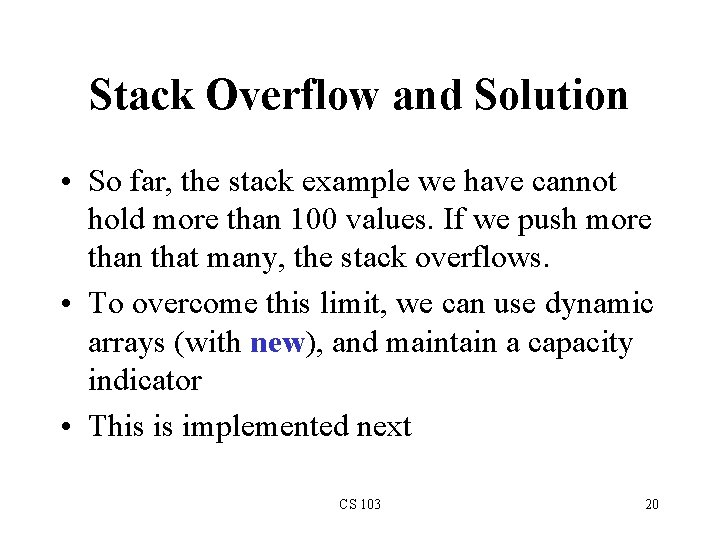Stack Overflow and Solution • So far, the stack example we have cannot hold more than 100 values. If we push more than that many, the stack overflows. • To overcome this limit, we can use dynamic arrays (with new), and maintain a capacity indicator • This is implemented next CS 103 20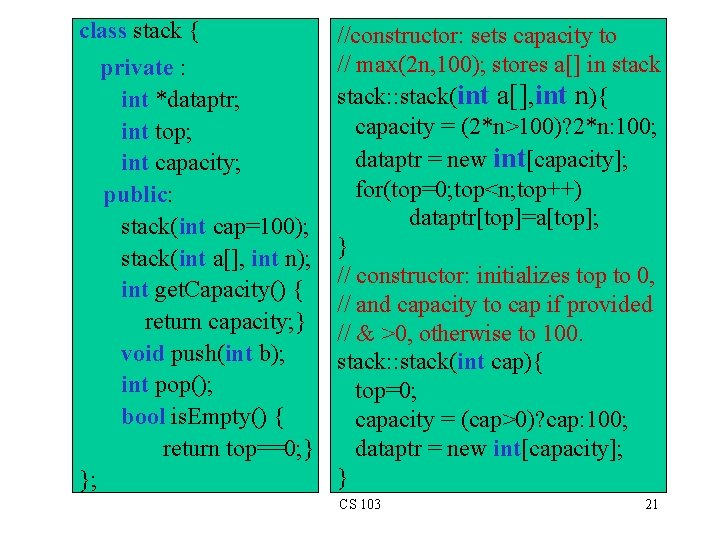class stack { //constructor: sets capacity to // max(2 n, 100); stores a[] in stack private : stack: : stack(int a[], int n){ int *dataptr; capacity = (2*n>100)? 2*n: 100; int top; dataptr = new int[capacity]; int capacity; for(top=0; top<n; top++) public: dataptr[top]=a[top]; stack(int cap=100); stack(int a[], int n); } // constructor: initializes top to 0, int get. Capacity() { // and capacity to cap if provided return capacity; } // & >0, otherwise to 100. void push(int b); stack: : stack(int cap){ int pop(); top=0; bool is. Empty() { capacity = (cap>0)? cap: 100; return top==0; } dataptr = new int[capacity]; } }; CS 103 21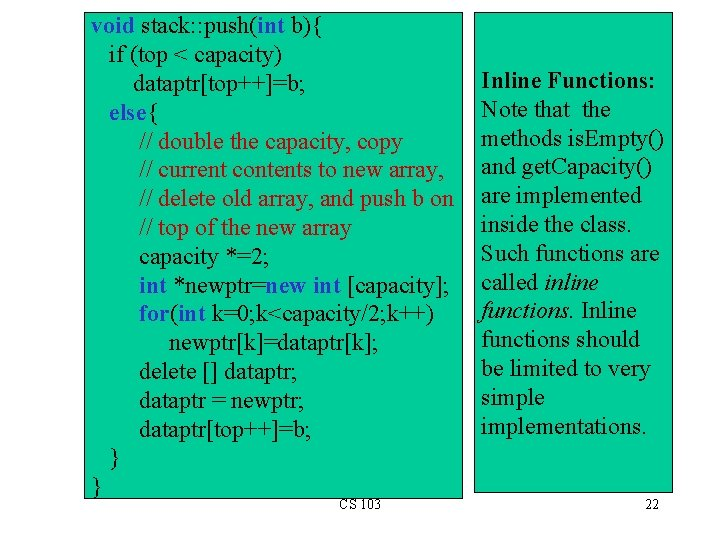void stack: : push(int b){ if (top < capacity) dataptr[top++]=b; else{ // double the capacity, copy // current contents to new array, // delete old array, and push b on // top of the new array capacity *=2; int *newptr=new int [capacity]; for(int k=0; k<capacity/2; k++) newptr[k]=dataptr[k]; delete [] dataptr; dataptr = newptr; dataptr[top++]=b; } } CS 103 Inline Functions: Note that the methods is. Empty() and get. Capacity() are implemented inside the class. Such functions are called inline functions. Inline functions should be limited to very simplementations. 22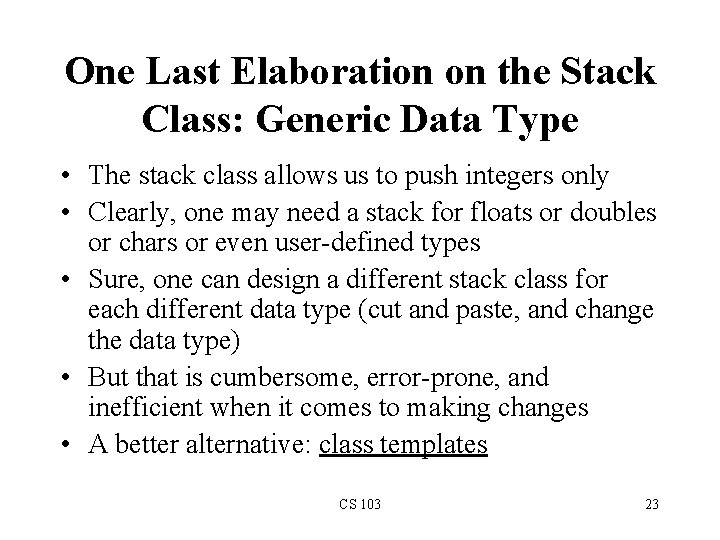One Last Elaboration on the Stack Class: Generic Data Type • The stack class allows us to push integers only • Clearly, one may need a stack for floats or doubles or chars or even user-defined types • Sure, one can design a different stack class for each different data type (cut and paste, and change the data type) • But that is cumbersome, error-prone, and inefficient when it comes to making changes • A better alternative: class templates CS 103 23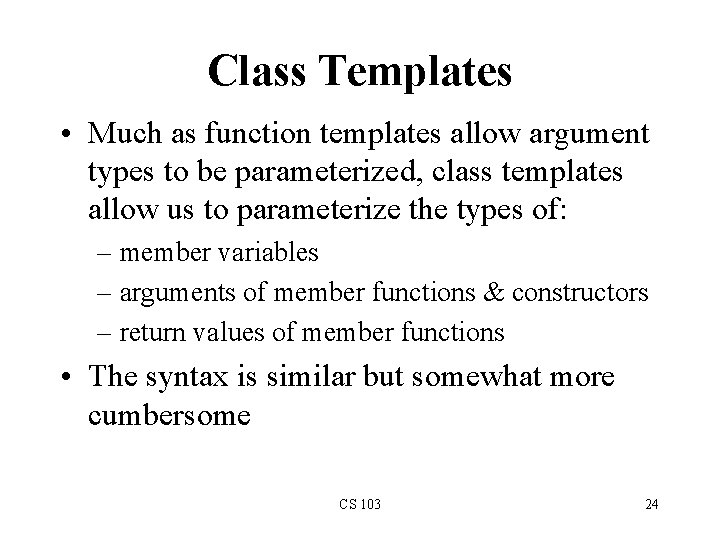Class Templates • Much as function templates allow argument types to be parameterized, class templates allow us to parameterize the types of: – member variables – arguments of member functions & constructors – return values of member functions • The syntax is similar but somewhat more cumbersome CS 103 24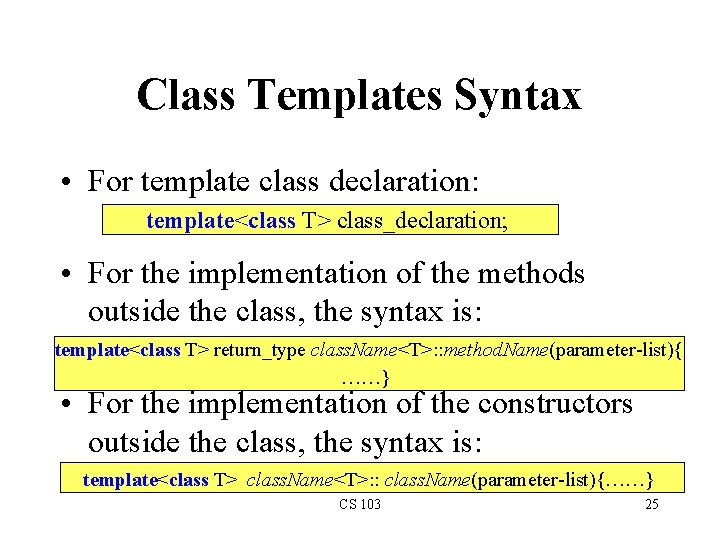Class Templates Syntax • For template class declaration: template<class T> class_declaration; • For the implementation of the methods outside the class, the syntax is: template<class T> return_type class. Name<T>: : method. Name(parameter-list){ ……} • For the implementation of the constructors outside the class, the syntax is: template<class T> class. Name<T>: : class. Name(parameter-list){……} CS 103 25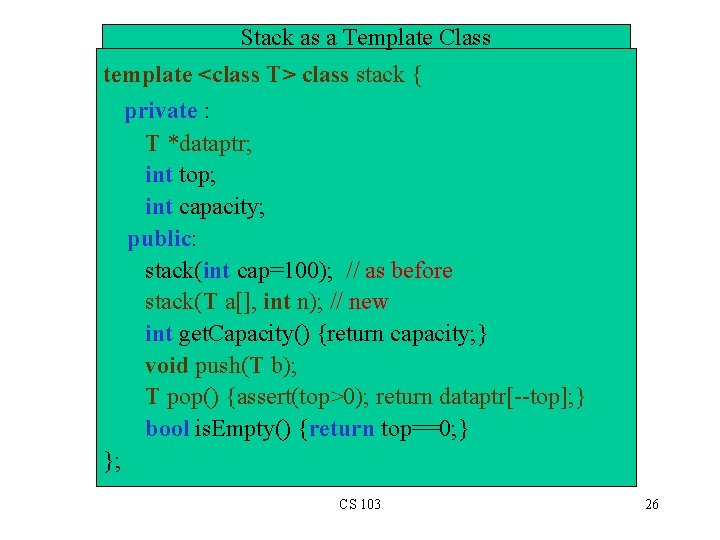Stack as a Template Class template <class T> class stack { private : T *dataptr; int top; int capacity; public: stack(int cap=100); // as before stack(T a[], int n); // new int get. Capacity() {return capacity; } void push(T b); T pop() {assert(top>0); return dataptr[--top]; } bool is. Empty() {return top==0; } }; CS 103 26//constructor: sets capacity to max(2 n, 100). // It then initializes stack to a[]. template<class T> stack<T>: : stack(T a[], int n){ capacity = (2*n>100)? 2*n: 100; dataptr = new T[capacity]; for(top=0; top<n; top++) dataptr[top]=a[top]; } // constructor: initializes top to 0, and capacity to cap // if provided & >0, otherwise to 100. template<class T> stack<T>: : stack(int cap){ top=0; capacity = (cap>0)? cap: 100; dataptr = new T[capacity]; } CS 103 27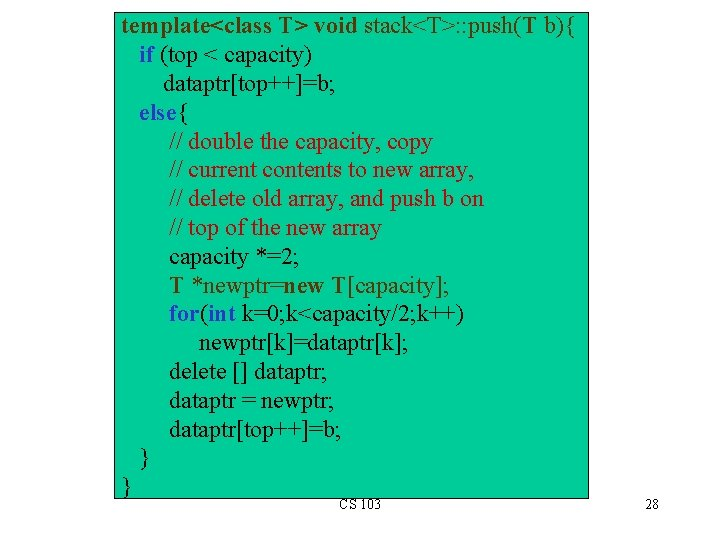template<class T> void stack<T>: : push(T b){ if (top < capacity) dataptr[top++]=b; else{ // double the capacity, copy // current contents to new array, // delete old array, and push b on // top of the new array capacity *=2; T *newptr=new T[capacity]; for(int k=0; k<capacity/2; k++) newptr[k]=dataptr[k]; delete [] dataptr; dataptr = newptr; dataptr[top++]=b; } } CS 103 28A Complete Program Using Template Stacks #include <cstdlib> #include <iostream> using namespace std; // template stack definition goes here int main(int argc, char *argv[]){ stack<int> int. S(5); // a stack of integers cout<<"int. S capacity after construction = "<<int. S. get. Capacity()<<endl; int x[]={2, 3, 7, 8, -10, 14, 5}; for (int i=0; i<7; i++) int. S. push(x[i]); cout<<"int. S capacity after pushing 7 elements="<< int. S. get. Capacity(); cout<<“n. Emptying int. S: "; while (!int. S. is. Empty()) cout<<int. S. pop()<<"; "; cout<<endl; CS 103 29stack<char *> string. S(5); // a stack of strings string. S. push("hi"); string. S. push("there"); cout<<"Emptying string. S: "; while (!string. S. is. Empty()) cout<<string. S. pop()<<"; "; cout<<endl; double y[]={3. 14, 9. 8, 1. 42, 12}; stack<double> double. S(y, 4); // a stack of doubles cout<<"double. S capacity="<<double. S. get. Capacity()<<endl; cout<<"Emptying double. S: "; while (!double. S. is. Empty()) cout<<double. S. pop()<<"; "; cout<<endl; } CS 103 30The Output of the Last Program int. S capacity after construction = 5 int. S capacity after pushing 7 elements=10 Emptying int. S: 5; 14; -10; 8; 7; 3; 2; Emptying string. S: there; hi; double. S capacity=100 Emptying double. S: 12; 1. 42; 9. 8; 3. 14; CS 103 31